# AP Calculus BC Practice Test 33

### Test Information9 questions18 minutes

Calculator Disallowed

1. Which of the following statements about the graph of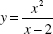is (are) true?

I. The graph has no horizontal asymptote.

II. The line x = 2 is a vertical asymptote.

III. The line y = x + 2 is an oblique asymptote.

2. The only function that does not satisfy the Mean Value Theorem on the interval specifiedis

3.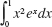4.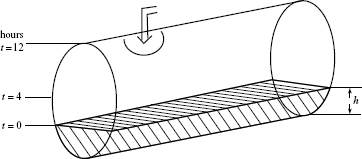A cylindrical tank, shown in the figure above, is partially full of water at timet = 0, when more water begins flowing in at a constant rate. The tank becomes halffull when t = 4, and is completely full when t = 12. Let h represent the height of the water at time t. During which interval is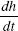increasing?

5.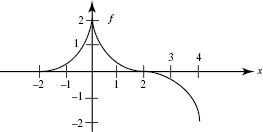The graph of function f shown above consists of three quarter-circles.

Which of the following is (are) equivalent to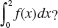I.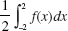II.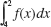III.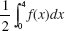6. The base of a solid is the first-quadrant region bounded by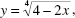and each cross section perpendicular to the x-axis is a semicircle with a diameter in the xy-plane. The volume of the solid is

7. The average value of f (x) = 3 + |x| on the interval [-2, 4] is

8. The area inside the circle r = 3 sin θ and outside the cardioid r = 1 + sin θ is given by

9. Let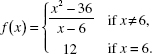Which of the following statements is (are) true?

I. f is defined at x = 6.

II.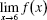exists.

III. f is continuous at x = 6.